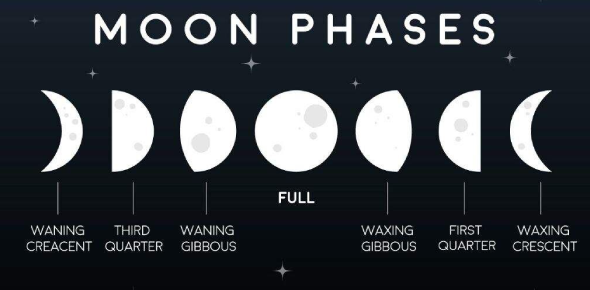# Quiz On The Phases Of Moon

10 Questions | Total Attempts: 2753SettingsComplete the following quiz. You must score at least an 80% or you will have to retake the quiz. All the best!

Related Topics
• 1.
The following is a picture of what moon phase?
• A.

Full

• B.

New

• C.

Gibbous

• D.

Crescent

• 2.
The following is a picture of what moon phase?
• A.

New

• B.

Full

• C.

Gibbous

• D.

Crescent

• 3.
The following is a picture of what moon phase?
• A.

Full

• B.

New

• C.

Gibbous

• D.

Crescent

• 4.
The following is a picture of what moon phase?
• A.

Quarter

• B.

Half

• C.

Gibbous

• D.

Crescent

• 5.
The following shows the moon phases are_____________________.
• A.

Waxing

• B.

Waning

• 6.
The following shows the moon phases are______________________.
• A.

Waxing

• B.

Waning

• 7.
Which Quarter Moon is shown below?
• A.

First Quarter

• B.

Second Quarter

• C.

Third Quarter

• D.

Fourth Quarter

• 8.
Which Quarter Moon is shown below?
• A.

First Quarter

• B.

Second Quarter

• C.

Third Quarter

• D.

Fourth Quarter

• 9.
The moon goes through all of its phases every____________________________.
• A.

10 days

• B.

15 days

• C.

29 days

• D.

60 days

• 10.
The following is a picture of what moon phase?
• A.

Full

• B.

New

• C.

Gibbous

• D.

Crescent